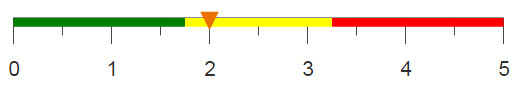# Finding missing values by linking tables

When merging tables, sometimes they do not have equal amounts of information. When using match="pad", the columns that do not have matches are populated with NA values for the foreign table. To find the missing values that are added when tables that have unmatched columns, you can create a reference or lookup table.

## Difficulty## Objective

You wanted to merge several tables together, but some tables contained more columns than others, so you used the <merge> operation with match="pad". The merged table now contains N/A values in its columns for those rows from the foreign tables that did not have a matching column. Based on common information between the tables that were merged together, you can determine the missing values by creating a reference table. This reference table can then be linked to your merged table to create a lookup column for the missing values. For example, if the foreign tables you are merging contained columns for the city and zip code, but your base table only contained a column for the city, you could fill in the missing zip code information based on the data in the foreign tables.

## Solution

```<base table="pub.doc.gov.area.x2003"/>
<willbe name="year" label="Year" value="2003" format="type:nocommas"/>
<merge table2="pub.doc.gov.area.x2004" match="pad">
<willbe name="year" label="Year" value="2004" format="type:nocommas"/>
</merge>
<link table2="pub.doc.gov.area.x2003" col="state,county"
col2="state,county" suffix="_lookup">
<colord cols="state,county,countyname"/>
</link>
<willbe name="county_name"
value="if(countyname=NA;countyname_lookup;countyname)"/>
<colord hide="countyname,countyname_lookup"/>```

## Alternate solution

```<base table="pub.doc.gov.county.x2002"/>
<merge table2="pub.doc.gov.county.x2003" match="pad"/>
<link table2="pub.doc.gov.county" col="state" col2="state"
suffix="_lookup">
<loop with_="alphnum">
<outer>
<directory folder="pub.doc.gov.county"/>
<colord cols="path"/>
<col name="path" format="width:50"/>
<transpose/>
</outer>
<inner>
<base table="{@alphnum.m2}"/>
<letseq tables="{@alphnum}" keys="{pkg_names(@tables)}">
<foreach table_year="{@keys}" tally_="@i">
<if test="{@i>3}">
<then>
<merge table2="{@alphnum.{@table_year}}"/>
</then>
<else/>
</if>
</foreach>
</letseq>
</inner>
</loop>
<colord cols="state,state_alpha"/>
<sel value="g_first1(state;;)"/>
</link>
<willbe name="statealpha"
value="if(state_alpha=NA;state_alpha_lookup;state_alpha)"/>
<colord hide="state_alpha,state_alpha_lookup"/>```

## Discussion

Often when merging tables, one table might have more columns, and therefore, more information, than another table. Thus, when you merge the tables, there will be rows that have missing values. This information can be found if the tables share other information that correlates to the missing values.

For example, in the first solution, tables containing two different years of area rent data are merged together. One table contains a column for the county number as well as a column for the county name, but the other table only contains a column for the county number. In the merged table, the column for the county name will contain N/A values for those rows from the table that did not have any county name information. By creating a reference column that shows how each number correlates with each name, the missing county names can be determined.

The first solution merges the tables using the <merge> operation with match="pad" to make sure that if the table columns don't match, all are still included in the merge. The <colord> operation is used to display only those columns that uniquely identify each county name in a new worksheet, which is then linked into the merged worksheet. By setting col and col2 equal to these identifying columns, a countyname_lookup column is created in the merged worksheet. A new column is then created that contains a full list of county names, by using the original name if it existed, or the lookup value if it was N/A.

If you are sure that the table you are using to create a reference column includes all possible values, then the first solution in this recipe works and uses a minimal amount of code. However, if the values are not all inclusive, it is a better idea to create a reference table using the information from multiple tables. In the recipe Combining rows of multiple tables, the <loop> operation was used to merge multiple tables together. Using this same idea, a reference table can be created by looping through the information of multiple tables within the <link> operation.

The second solution merges rows from two tables of county rent data. One table contains a column for the state number and the state abbreviation, while the other table only contains the state number. To make sure there is a reference for every possible state, this solution loops through all of the years of county rent data to create an all-inclusive reference table. As in the first solution, the reference table is then linked into the merged table to find the missing values.

## Further reading

If you would like to learn more about the functions and operations discussed in this recipe, click on the links below:

<merge>

<link>

if(C1;R1;C2;R2;...;D)